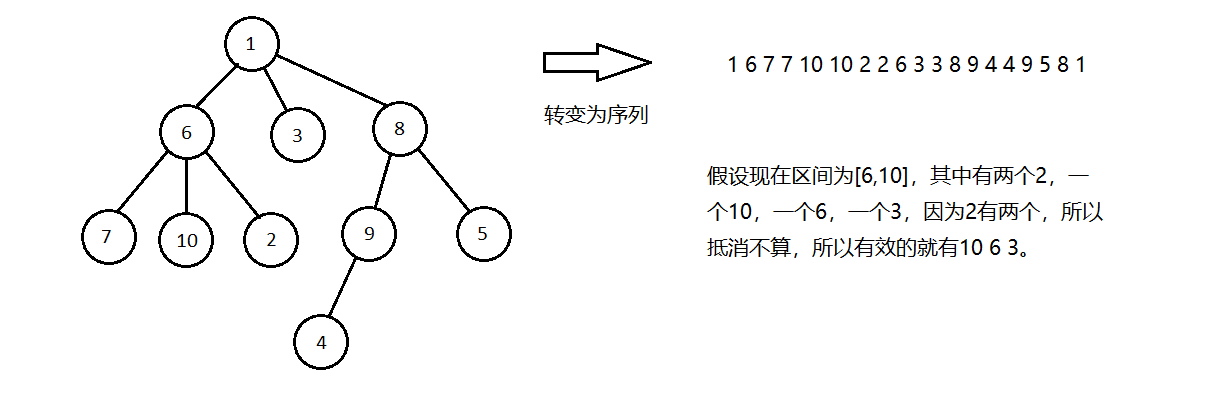# 莫队浅谈&题目讲解

### 一、莫队的思想以及莫队的前置知识

莫队是一种离线的算法，他的实现借用了分块的思想。在学习莫队之前，本人建议学习一下分块，并对其有一定的理解。

### 二、莫队

现给出一道例题：bzoj2038 ，首先这一道题可以转化为询问一个区间，$\sum \limits_{i=1}^{n} many[i]\times(many[i]-1)$，$many[i]$表示颜色$i$在区间$[l,r]$中的个数。

我们先考虑暴力，我们对于每一个询问进行$O(n)$的颜色统计，用桶统计出来每一种颜色的个数，我们用$O(n)$扫一下整个桶，求出答案即可。

现在考虑我们要讲解的知识点，对于莫队，询问要有一个必要的条件，所有的询问必须是离线的，因为我们要对所有的询问进行一个排序。我们维护两个指针$l,r$，这两个指针表示当前我们已经统计的区间，即在区间$[l,r]$中的所有颜色都已经记录在桶中了，现在考虑两个询问之间的维护，假设我们现在已经处理完询问$[a,b]$，现在要处理询问$[c,d]$，我们将指针$l,r$在序列上暴力跳动，使指针$l$从$a$到$c$，指针$r$从$b$到$d$，这样我们就能用$O((|c-a|+|d-b|)\times 一次修改时间)$来进行两个询问之间的更改。

我们考虑指针的移动，一共有四种情况：$l$的左移，$l$的右移，$r$的左移，$r$的右移。先考虑$l$的右移，我们先移动指针，这样就需要将$l-1$这个位置的颜色的贡献减去，考虑$l-1$位置的颜色对答案贡献的改变，从$many[col[l-1]]^2$，变成$(many[col[l-1]]-1)^2$，处理一下其中的$\Delta$就好了，不要忘记最后$many[col[l-1]]--$。考虑$r$指针的左移，他和$l$指针的右移是一个道理，只不过是将$r+1$位置的颜色删掉。考虑$l$指针的左移，不同于右移的是，这回是加，我们先移动指针，这样我们就是要将$col[l]$加入到贡献里，$col[l]$对答案的贡献从$many[col[l]]^2$，变为了$(many[col[l]]+1)^2$，最后不要忘记$many[col[l]]++$。考虑$r$指针的右移，他和$l$的左移是一个道理，只不过是将$++r$位置的数字添加进去贡献。

我们现在知道一个区间的信息，想知道另一个就只需要$O(左端点距离+右端点距离)$。但是这样我们的算法还是不够优秀，我们考虑将前面的排序提到日程上来，我们考虑一个十分优秀的排序方式使得我们时间复杂度最优，我们定义一个$Siz$，使其等于$\sqrt n$，我们按照$Siz$进行分块，我们定义排序的第一关键字，询问区间$l$所在块的编号，第二关键字，询问$r$的大小，这样我们就能得到一个十分优秀的时间复杂了，我们分析一下，因为$l$在同一个块中的所有询问的右端点是按照从小到大的顺序排列的，所以对于$l$在一个块中的所有询问，我们的$r$指针最多会从$1$遍历到$n$，一共有$\sqrt n$个块，$r$指针一共就只会移动$O(n\sqrt n)$次。我们现在考虑$l$指针，我们每一个询问都只会在所在块中移动，所以还是$O(n\sqrt n)$的，所以最终的中时间复杂度就是$O(n\sqrt n)$。

#include <cmath>
#include <cstdio>
#include <algorithm>
using namespace std;
#define N 100010
int Siz,n,m,num[N],many[N],l,r;long long now,ans[N];
int bel(int x) {return x/Siz+(x%Siz!=0);}
{return bel(a.l)<bel(b.l)||(bel(a.l)==bel(b.l)&&a.r<b.r);}
{now-=1ll*many[x]*(many[x]-1),many[x]++,now+=1ll*many[x]*(many[x]-1);}
void del(int x)
{now-=1ll*many[x]*(many[x]-1),many[x]--,now+=1ll*many[x]*(many[x]-1);}
int main()
{
scanf("%d%d",&n,&m),Siz=sqrt(n);
for(int i=1;i<=n;i++) scanf("%d",&num[i]);
for(int i=1;i<=m;i++)
{
}
for(int i=1;i<=m;i++)
{
tmp*=(tmp-1);
if(!ans[i]) printf("%d/%d\n",0,1);
else printf("%lld/%lld\n",ans[i]/__gcd(ans[i],tmp),tmp/__gcd(tmp,ans[i]));
}
}


### 三、莫队

bzoj3781 小B的询问

这道题与例题所求基本一致，我们只需要用莫队算法维护区间中每一中数字出现的次数，并动态维护出来题目中要求的和就好了。

bzoj3585 mex

这道题是求区间$mex$，我们考虑用莫队维护每一个区间的数字出现次数，当然也是用桶，我们现在考虑哪些数字能对答案进行贡献，即数字小于等于$n$的数字才会有贡献，所以我们只需要记录小于等于$n$的数字即可。但是我们知道了每一个数字出现的次数，怎么去找$mex$？我们门考虑分块，我们对数字进行分块，我们考虑维护一个数组$have[i]$，表示数字所分的第$i$个块有多少个数字出现过，这个数组是在莫队的时候维护的，在例题中我们对答案赋值的位置就要更改成为一个函数，在这个函数中我们合理运用分块的思路，我们用$\sqrt n$的时间判断$\sqrt n$个块是不是都是满的，即$have[i]=Siz$，找到第一个不是满的，$\sqrt n$的时间便利和这个块，找到第一个没出现数字即可，时间复杂度$n\sqrt n+m\sqrt n$。

bzoj3809 Gty的二逼妹子序列&&bzoj3236 作业

我们考虑上一道题的思路，我们依旧按照权值分块，还是每一个块统计出一个$have[i]$，表示数字所分的第$i$个块有多少个数字出现过，考虑求答案，对于权值所分的块的整块的，直接加上$have[i]$，零散的我们暴力就好了，一共的时间复杂度依旧是$n\sqrt n+m\sqrt n$。

当然我们这道题还可以在莫队上套树状数组，对于每一个新出现的数字，在树状数组对应位置上加一，对于消失的一个数字，在树状数组对应位置上减一，每一次就是求一个区间的和，我的这个写法时间超限了，时间复杂度$O(n\sqrt n log_2^n)$。并没有那么优秀。

bzoj3289 Mato的文件管理

我们考虑这道题的本质，每一次给出一个区间$[l,r]$，求$[l,r]$内所有数字组成的逆序对个数。依旧考虑莫队，这道题不像上面的题是用桶来维护出现次数，我们运用树状数组。

考虑移动，每一次在后面添加一个数字，比这个数大的数都会与其形成一个逆序对，用树状数组查一下，加上。每次在最后移除一个数，比这个数大的数都会与其形成一个逆序对，用树状数组查一下，减去。每次在最前添加一个数，比这个数小的数都会与其减少一个逆序对，用树状数组查一下，加上。每次在最前移除一个数，比这个数小的数都会与其减少一个逆序对，用树状数组查一下，减去。

### 三、带修改莫队

带修改莫队的思路与莫队的思路相同，都是根据分块的思想进行排序，只不过带修改莫队还会多一个指针$t$，表示时间，这个指针表示当前我们的修改修改到了哪里，对于每一个修改，我们定义四个值：分别为修改时间，修改位置，修改前的内容，修改后的内容。对于每一个询问，我们多定义一个值，表示他前面的最后一个修改的时间。

我们将排序更改一下，在原本的两个关键字后再加入一个关键字，即时间，判断条件时，当两个询问的区间的左端点在一个块中，右端点在一个块中，我们将这两个询问按照时间排序。我们对于原本的莫队循环要进行一定的修改，因为我们还有一个指针叫做时间，我们需要在调整区间的两个指针之前调整好时间的指针，这样我们就可以进行操作了。

我们考虑上面的讲解，我们将这个颜色序列复制下来，计复制得到的序列为$change$，我们在$change$上做所有修改操作，这样我们就能得到每一个修改操作的四个值（修改时间，修改位置，修改前的内容，修改后的内容）。这样我们按照上面的思路进行书写就可以了。

我们发现如果把$Siz=\sqrt n$你会跑的很慢，所以我们考虑更改一下我们的$Siz$大小，我们考虑将$Siz$变成$n^{\frac{2}{3}}$，这样我们再来分析一下时间复杂度。

对于时间的移动，对于左右端点所在块不变的情况，时间是单调向右移的，总共$O(n)$，左右端点之一所在块改变，时间最多从$n$直接移回$1$，复杂度$O(n)$，左右端点所在块各有$O(n^\frac{1}{3})$种，两两组合有$O(n^\frac{2}{3})$种，每种都是$O(n)$，总复杂度是$O(n^\frac{5}{3})$。

对于右端点的移动，在左右端点所在块不变时，每次最多移动$n^\frac{2}{3}$，一共最多有$n$次右端点的移动，复杂度是$O(n^\frac{5}{3})$，当左端点所在块改变时，右端点最多从$n$一直移动到$1$，距离是$n$，最多有$n^\frac{2}{3}$次这样的移动，复杂度是$O(n^\frac{4}{3})$；总共右端点移动的复杂度是$O(n^\frac{5}{3})$。

对于左端点的移动，在左端点块不变时，一次移动距离最多$O(n^\frac{2}{3})$，总共$O(n^\frac{5}{3})$。而跨块时，由于左端点所在块是单调向右移动的，复杂度最大的情况就是每跨一个块都是从前一个块的最左侧跑到后一个块的最右侧，距离$O(n^\frac{1}{3})$，总复杂度$O(n)$。所以总共左端点移动的复杂度是$O(n^\frac{5}{3})$。

一般情况下，带修改莫队都会和树上莫队联系在一起出题。

#include <cstdio>
#include <algorithm>
using namespace std;
#define N 1000010
#define Siz 330
int n,m,l,r,t,idx,cnt,many;
int chan[N],num[N],bin[N],ans[N];
struct Oper {int pla,num,old;}oper[N];
{
}
void del(int x) {--bin[x];if(bin[x]==0) many--;}
void change(int x,int y) {if(x<=r&&x>=l) add(y),del(num[x]);num[x]=y;}
int main()
{
scanf("%d%d",&n,&m);
for(int i=1;i<=n;i++) scanf("%d",&num[i]),chan[i]=num[i];
for(int i=1;i<=m;i++)
{
char kind;scanf("%s",kind);
else ++cnt,scanf("%d%d",&oper[cnt].pla,&oper[cnt].num),
oper[cnt].old=chan[oper[cnt].pla],chan[oper[cnt].pla]=oper[cnt].num;
}
for(int i=1;i<=idx;i++)
{
}
for(int i=1;i<=idx;i++) printf("%d\n",ans[i]);
}


### 四、树上莫队

树上莫队一共有两种，一种是求树上子树信息，另一种是求树上路径信息，这两种都能从树上转成序列上。

我们先考虑第一种，我们考虑一个叫$dfs$序的东西，我们可以将求子树信息，转化为求$dfs$序的一段区间的信息，直接上序列上的莫队就可以了。

考虑第二种，我们考虑一个叫入栈出栈序的东西，我们这样能得到一个长度为$2n$的序列。现在假设我们统计的区间为$[l,r]$，即两个指针所夹的闭区间。在这个区间里，若有两个相同的编号，即有一个编号的入栈出栈序都在这个统计区间里，我们认为他们两个相互抵消了。如下图：我们观察上图，我们能发现一个问题，我们将路经询问分为两类，一类是两个点为祖先关系，这样我们直接另询问区间为祖先的出栈序到另一个点的入栈序，这个区间去掉抵消掉的，就刚刚好好是我们的路径。另一类是这两个点不为祖先关系，我们另询问区间是一个点的出栈序，到另一点的入栈序，若不为区间，即$r\lt l$，我们交换两个点，我们得到的这个区间不难发现出去抵消掉的点之后，剩下的点就是路径上的点，但不包括$lca$，这样我们在记录答案的时候在吧$lca$的贡献加进去就好了，具体看代码，画画图就好了。

我们讲题目所求得转化一下，因为没有人想做回头路，所以就是询问一棵树上两个点之间的简单路径的糖果的个数$\times$一定的权值。当然还有一个操作是修改，即带修改树上莫队。这道题目十分简单，他就是简单的带修改莫队加上树上莫队。

#include <cmath>
#include <cstdio>
#include <algorithm>
using namespace std;
#define N 200010
struct Oper {int pla,to,old;}oper[N];
int place[N],fa[N],col[N],chan[N];
int n,m,q,idx,cnt,tot,in[N],out[N],l,r,t;
long long now,ans[N]; int level[N]; bool is[N];
void dfs(int p,int from)
{
in[p]=++cnt,place[cnt]=p;
// printf("%d %d\n",p,cnt);
fa[p]=from,level[p]=level[from]+1;
dfs(to[i],p);
out[p]=++cnt,place[cnt]=p;
}
int find_lca(int x,int y)
{
if(level[x]>level[y]) swap(x,y);
for(int i=19;~i;i--) if((1<<i)<=level[y]-level[x])
y=fa[i][y];
if(x==y) return x;
for(int i=19;~i;i--) if(fa[i][x]!=fa[i][y])
x=fa[i][x],y=fa[i][y];
return fa[x];
}
{
}
void change(int x,int y)
{
int tmp=num1[col[x]],tmp2=num1[y];
if(in[x]<=r&&in[x]>=l) if(is[x])
now-=1ll*tmp*num2[many[col[x]]],many[col[x]]--,
many[y]++,now+=1ll*tmp2*num2[many[y]];
if(out[x]<=r&&out[x]>=l) if(is[x])
now-=1ll*tmp*num2[many[col[x]]],many[col[x]]--,
many[y]++,now+=1ll*tmp2*num2[many[y]];
col[x]=y;
}
{
if(is[x]) now-=1ll*num1[col[x]]*num2[many[col[x]]],many[col[x]]--,is[x]=false;
else many[col[x]]++,now+=1ll*num1[col[x]]*num2[many[col[x]]],is[x]=true;
}
void del(int x)
{
if(is[x]) now-=1ll*num1[col[x]]*num2[many[col[x]]],many[col[x]]--,is[x]=false;
else many[col[x]]++,now+=1ll*num1[col[x]]*num2[many[col[x]]],is[x]=true;
}
int main()
{
scanf("%d%d%d",&n,&m,&q),Siz=pow(n,0.66666);
for(int i=1;i<=m;i++) scanf("%d",&num1[i]);
for(int i=1;i<=n;i++) scanf("%d",&num2[i]);
dfs(1,0),cnt=0;
for(int i=1;i<=19;i++) for(int j=1;j<=n;j++)
fa[i][j]=fa[i-1][fa[i-1][j]];
for(int i=1;i<=n;i++) scanf("%d",&col[i]),chan[i]=col[i];
for(int i=1,type,x,y;i<=q;i++)
{
scanf("%d%d%d",&type,&x,&y);
if(type==0) ++cnt,oper[cnt].pla=x,oper[cnt].to=y;
else
{
int lca=find_lca(x,y);
if(in[x]>in[y]) swap(x,y);
}
}
for(int i=1;i<=cnt;i++)
oper[i].old=chan[oper[i].pla],chan[oper[i].pla]=oper[i].to;
for(int i=1;i<=tot;i++)
{